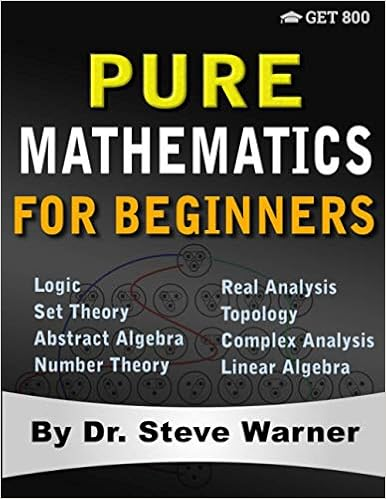# Pure Mathematics for Beginners: A Rigorous Introduction to Logic, Set Theory, Abstract Algebra, Number Theory, Real Analysis, Topology, Complex Analysis, and Linear Algebra

#### Tag: Mathematics

Posted on 2020-11-16, by temrick.

DescriptionPure Mathematics for Beginners consists of a series of lessons in Logic, Set Theory, Abstract Algebra, Number Theory, Real Analysis, Topology, Complex Analysis, and Linear Algebra. The 16 lessons in this book cover basic through intermediate material from each of these 8 topics. In addition, all the proofwriting skills that are essential for advanced study in mathematics are covered and reviewed extensively. Pure Mathematics for Beginners is perfect for
• professors teaching an introductory college course in higher mathematics
• high school teachers working with advanced math students
• students wishing to see the type of mathematics they would be exposed to as a math major.
The material in this pure math book includes:
• 16 lessons in 8 subject areas.
• A problem set after each lesson arranged by difficulty level.
• A complete solution guide is included as a downloadable PDF file.
Lesson 1 - Logic: Statements and Truth
Lesson 2 - Set Theory: Sets and Subsets
Lesson 3 - Abstract Algebra: Semigroups, Monoids, and Groups
Lesson 4 - Number Theory: Ring of Integers
Lesson 5 - Real Analysis: The Complete Ordered Field of Reals
Lesson 6 - Topology: The Topology of R
Lesson 7 - Complex Analysis: The Field of Complex Numbers
Lesson 8 - Linear Algebra: Vector Spaces
Lesson 9 - Logic: Logical Arguments
Lesson 10 - Set Theory: Relations and Functions
Lesson 11 - Abstract Algebra: Structures and Homomorphisms
Lesson 12 - Number Theory: Primes, GCD, and LCM
Lesson 13 - Real Analysis: Limits and Continuity
Lesson 14 - Topology: Spaces and Homeomorphisms
Lesson 15 - Complex Analysis: Complex Valued Functions
Lesson 16 - Linear Algebra: Linear Transformations

https://nitroflare.com/view/6BE68C56AF836EC/0999811757.pdf

6892 dl's @ 3992 KB/s
5827 dl's @ 2985 KB/s
8063 dl's @ 3081 KB/s

Search More...
Pure Mathematics for Beginners: A Rigorous Introduction to Logic, Set Theory, Abstract Algebra, Number Theory, Real Analysis, Topology, Complex Analysis, and Linear AlgebraDownload links for "Pure Mathematics for Beginners: A Rigorous Introduction to Logic, Set Theory, Abstract Algebra, Number Theory, Real Analysis, Topology, Complex Analysis, and Linear Algebra":

Related Books